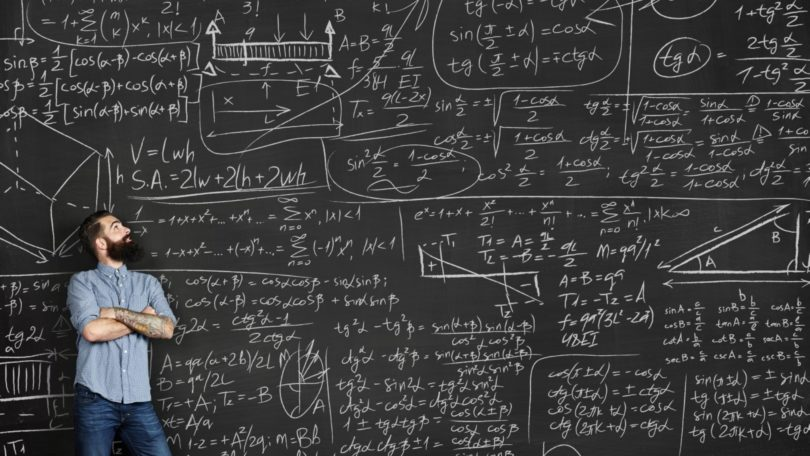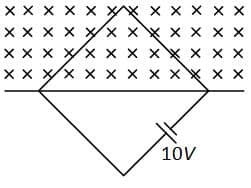Engineering Entrance Sample PapersGalgotias Engineering Entrance Examination physics sample paper with 30 questions as per GEEE Entrance exam pattern.

(a) Irreversible process
(b) Self disintegration process
(c) Spontaneous process
(d) All of the above
Ans. (d)

Ques. According to Bohr’s theory of hydrogen atom, the angular momentum of an electron in any orbit of hydrogen atom is
(a) Directly proportional to the radius of the orbit
(b) Inversely proportional to the radius of the orbit
(c) Directly proportional to the square of the radius of the orbit
(d) Directly proportional to the square root of the radius of the orbit
Ans. (d)

Ques. When an object in placed at a distance of 25 cm from a mirror, the magnification is m1 The object is moved 15 cm away with respect to the earlier position, magnification becomes m2. If m1/m2 = 4, the focal length of the mirror is
(a) 10 cm
(b) 30 cm
(c) 15 cm
(d) 20 cm
Ans. (d)

Ques. The number of wavelengths in the visible spectrum
(a) 4000
(b) 6000
(c) 2000
(d) Infinite
Ans. (d)

Related: JEE Mains Physics questions

Ques. A Square loop of side 1m is placed in a perpendicular magnetic field. Half of the area of the loop lies inside the magnetic field. A battery of e.m.f. 10V and negligible internal resistance is connected in the loop. The magnetic field change with time according to relation B = 0.01 – 2t tesla. The total e.m.f. of the battery will be(a) 1 V
(b) 11 V
(c) 10 V
(d) 9 V
Ans. (d)

Ques. A convex mirror has a focal length f. A real object is placed at a distance f in front of it from the pole produces an image at
(a) Infinity
(b) f
(c) f/2
(d) 2f
Ans. (c)

Ques. If the focal lengths of objective and eye lens of a microscope are 1.2 cm and 3 cm respectively and the object is put 1.25 cm away from the objective lens and the final image is formed at infinity, then magnifying power of the microscope is
(a) 150
(b) 200
(c) 250
(d) 400
Ans. (b)

Ques. A condenser is charged to a potential difference of 200 Volt as a result of which it gains charge of 0.1 coulomb. When it is discharged then the energy released will be
(a) 2 Joule
(b) 10 Joule
(c) 1 Joule
(d) 20 Joule
Ans. (b)

Ques. The focal length of the objective and the eyepiece of a microscope are 4 mm and 25 mm respectively. If the final image is formed at infinity and the length of the tube is 16 cm the magnifying power of the microscope will be
(a) – 327. 5
(b) – 32.57
(c) 3.275
(d) 32.75
Ans. (a)

Related: JEE Mains Mathematics paper

Ques. The centre of the diffraction pattern formed by a circular disc is always
(a) Bright
(b) Dark
(c) Sometimes bright and sometimes dark depending on distance of screen
(d) Sometimes bright and sometimes dark depending upon the size of the disc
Ans. (a)

Ques. An ammeter is connected in series with a silver voltameter for calibration. By passing a certain amount of current for 30 minutes the mass of silver deposited is 1.0062 g and ammeter reading is 0.52A. The error in ammeter reading is
(a) +0.02A
(b) +0.04A
(c) –0.02A
(d) –0.04A
Ans. (a)

Ques. In biprism experiment the light source is
(a) Extended
(b) Narrow
(c) Multichromatic
(d) All of above
Ans. (b)

Ques. One end of a steel pipe of length 100m is hammered and a person standing at the other end hears two sound waves one through the air column and other through the material of the pipe. If the Young’s modulus of elasticity of steel is 2.0 x 1011 N/m2 and relative density is 7.5, then the time interval between these sound will be
(a) 0.284 s
(b) 2.82 s
(c) 0.028 s
(d) 2 s
Ans. (a)

Ques. Water is flowing with a velocity of 3m/s in a pipe of diameter 4 cm. This water enters another tube of diameter 2 cm. The velocity of water in this tube is
(a) 12 m/s
(b) 6 m/s
(c) 3 m/s
(d) 1.5 m/s
Ans. (a)

Ques. A tuning fork is sounded with vibrating strings of lengths 0.95m and 1m. It produces 6 beats with each one. The frequency of the tuning fork will be
(a) 150 Hz
(b) 200 Hz
(c) 225 Hz
(d) 234 Hz
Ans. (d)

Ques. A stress of 1 kg / mm2 is applied on a wire. If the modulus of elasticity of the wire is 1012 dyne/cm2, then the percentage increase in the length of the wire will be
(a) 0.007
(b) 0.0098
(c) 98
(d) 9.8
Ans. (b)

Ques. A closed organ pipe is in unision with a vibrating tuning fork of frequency 264 Hz. If the velocity of sound in air is 330 m/s, then the length of air column will be
(a) 100 cm
(b) 62.50 cm
(c) 93.75 cm
(d) 31.25 cm
Ans. (d)

Ques. The thermions are
(a) Protons
(b) Electrons
(c) Photons
(d) Positrons
Ans. (a)

Ques. In the interference pattern, energy is
(a) Created at the position of maxima
(b) Destroyed at the position of minima
(c) Conserved but is redistributed
(d) None of the above
Ans. (c)

Ques. For proper ventilation of building, windows must be open near the bottom and top of the walls so as to let pass
(a) In more air
(b) In cool air near the bottom and hot air out near the roof
(c) In hot air near the roof and cool air out near the bottom
(d) Out hot air near the roof
Ans. (b)

Ques. A plane mirror reflects a pencil of light to form a real image. Then the pencil of light incident on the mirror is
(a) Parallel
(b) Convergent
(c) Divergent
(d) None of the above
Ans. (b)

Ques. The energy gap in an intansic semiconductor is of the order of
(a) 0.1 eV
(b) 1 eV
(c) 5 eV
(d) 10 eV
Ans. (b)

Ques. A bus and a jeep are moving with same kinetic energy. Equal retarding forces are applied to both with the help of brakes. The distances covered by them before coming to rest will be
(a) More by jeep
(b) More by bus
(c) Equal by both
(d) Dependent upon the mass of jeep or bus
Ans. (c)

Ques. In a junction diode the direction of diffusion current is
(a) From P-region to N-region
(b) From N-region to P-region
(c) From P-region to N-region if the junction is forward biased and vice versa if junction is reverse biased
(d) From N-region to P-region if the junction is forward biased and vice versa if it is reverse biased
Ans. (a)

Ques. A biconvex lens forms a real image of an object placed perpendicular to its principal axis. Suppose the radii of curvature of the lens tend to infinity. Then the image would
(a) Disappear
(b) Remain as real image still
(c) Be virtual and of the same size as the object
(d) Suffer from aberrations
Ans. (c)

Ques. In a nuclear reactor cadmium rods are used for
(a) Neutron emission
(b) Absorption unnecessary neutrons
(c) Absorption of heat
(d) Reducing the velocity of neutrons
Ans. (b)

Ques. Semiconductor is damaged by the strong current due to
(a) Lack of free electron
(b) Excess of electrons
(c) Excess of proton
(d) None of these
Ans. (b)

Ques. In a discharge tube at 0.02 mm, there is a formation of
(a) FDS
(b) CDS
(c) Both space
(d) None of these
Ans. (b)

Ques. To get three images of a single object, one should have two plane mirrors at an angle of
(a) 30°
(b) 60°
(c) 90°
(d) 150°
Ans. (c)

Ques. Quantam nature of light is explained by which of the following phenomenon
(a) Huygen wave theory
(b) Photoelectric effect
(c) Maxwell electromagnetic theory
(d) de-Broglie theory
Ans. (b)

Ques. An artificial satellite moving in a circular orbit around the earth has a total (kinetic + potential) energy Eo. Its potential energy is
(a) –Eo
(b) 1.5 Eo
(c) 2 Eo
(d) Eo
Ans. (c)